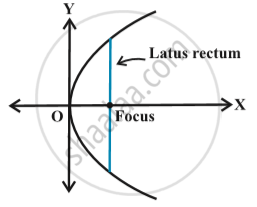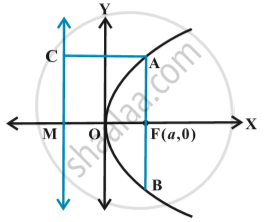Share

# Parabola - Latus Rectum

#### definition

Latus rectum of a parabola is a line segment perpendicular to the axis of the parabola, through the focus and whose end points lie on the parabola in following fig.To find the Length of the latus rectum of the parabola y^2 = 4ax in following fig.By the definition of the parabola, AF = AC.
But AC = FM = 2a
Hence AF = 2a.
And since the parabola is symmetric with respect to x-axis AF = FB and so
AB = Length of the latus rectum = 4a.

### Shaalaa.com

Latus rectum of parabola [00:01:58]
S
0%

S